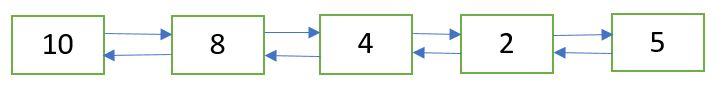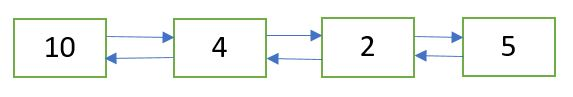Open In App

# Delete a Doubly Linked List node at a given position

Given a doubly linked list and a position n. The task is to delete the node at the given position n from the beginning.Doubly Linked List after deletion of node at position n = 2Approach: Following are the steps:

1. Get the pointer to the node at position n by traversing the doubly linked list up to the nth node from the beginning.
2. Delete the node using the pointer obtained in Step 1. Refer this post.

## C++

 `/* C++ implementation to delete a doubly Linked List node``   ``at the given position */``#include ` `using` `namespace` `std;` `/* a node of the doubly linked list */``struct` `Node {``    ``int` `data;``    ``struct` `Node* next;``    ``struct` `Node* prev;``};` `/* Function to delete a node in a Doubly Linked List.``   ``head_ref --> pointer to head node pointer.``   ``del  -->  pointer to node to be deleted. */``void` `deleteNode(``struct` `Node** head_ref, ``struct` `Node* del)``{``    ``/* base case */``    ``if` `(*head_ref == NULL || del == NULL)``        ``return``;` `    ``/* If node to be deleted is head node */``    ``if` `(*head_ref == del)``        ``*head_ref = del->next;` `    ``/* Change next only if node to be deleted is NOT``       ``the last node */``    ``if` `(del->next != NULL)``        ``del->next->prev = del->prev;` `    ``/* Change prev only if node to be deleted is NOT``       ``the first node */``    ``if` `(del->prev != NULL)``        ``del->prev->next = del->next;` `    ``/* Finally, free the memory occupied by del*/``    ``free``(del);``}` `/* Function to delete the node at the given position``   ``in the doubly linked list */``void` `deleteNodeAtGivenPos(``struct` `Node** head_ref, ``int` `n)``{``    ``/* if list in NULL or invalid position is given */``    ``if` `(*head_ref == NULL || n <= 0)``        ``return``;` `    ``struct` `Node* current = *head_ref;``    ``int` `i;` `    ``/* traverse up to the node at position 'n' from``       ``the beginning */``    ``for` `(``int` `i = 1; current != NULL && i < n; i++)``        ``current = current->next;` `    ``/* if 'n' is greater than the number of nodes``       ``in the doubly linked list */``    ``if` `(current == NULL)``        ``return``;` `    ``/* delete the node pointed to by 'current' */``    ``deleteNode(head_ref, current);``}` `/* Function to insert a node at the beginning``   ``of the Doubly Linked List */``void` `push(``struct` `Node** head_ref, ``int` `new_data)``{``    ``/* allocate node */``    ``struct` `Node* new_node =``         ``(``struct` `Node*)``malloc``(``sizeof``(``struct` `Node));` `    ``/* put in the data  */``    ``new_node->data = new_data;` `    ``/* since we are adding at the beginning,``    ``prev is always NULL */``    ``new_node->prev = NULL;` `    ``/* link the old list of the new node */``    ``new_node->next = (*head_ref);` `    ``/* change prev of head node to new node */``    ``if` `((*head_ref) != NULL)``        ``(*head_ref)->prev = new_node;` `    ``/* move the head to point to the new node */``    ``(*head_ref) = new_node;``}` `/* Function to print nodes in a given doubly``   ``linked list */``void` `printList(``struct` `Node* head)``{``    ``while` `(head != NULL) {``        ``cout << head->data << ``" "``;``        ``head = head->next;``    ``}``}` `/* Driver program to test above functions*/``int` `main()``{``    ``/* Start with the empty list */``    ``struct` `Node* head = NULL;` `    ``/* Create the doubly linked list 10<->8<->4<->2<->5 */``    ``push(&head, 5);``    ``push(&head, 2);``    ``push(&head, 4);``    ``push(&head, 8);``    ``push(&head, 10);` `    ``cout << ``"Doubly linked list before deletion:n"``;``    ``printList(head);` `    ``int` `n = 2;` `    ``/* delete node at the given position 'n' */``    ``deleteNodeAtGivenPos(&head, n);` `    ``cout << ``"\nDoubly linked list after deletion:n"``;``    ``printList(head);` `    ``return` `0;``}`

## Java

 `/* Java implementation to delete a``   ``doubly Linked List node``   ``at the given position */``   ` `// A node of the doubly linked list``class` `Node``{``    ``int` `data;``    ``Node next, prev;``}` `class` `GFG``{``    ``static` `Node head = ``null``;``    ``// Function to delete a node``    ``// in a Doubly Linked List.``    ``// head_ref --> pointer to head node pointer.``    ``// del --> pointer to node to be deleted.``    ``static` `Node deleteNode(Node del)``    ``{``        ``// base case``        ``if` `(head == ``null` `|| del == ``null``)``            ``return` `null``;` `        ``// If node to be deleted is head node``        ``if` `(head == del)``            ``head = del.next;` `        ``// Change next only if node to be``        ``// deleted is NOT the last node``        ``if` `(del.next != ``null``)``            ``del.next.prev = del.prev;` `        ``// Change prev only if node to be``        ``// deleted is NOT the first node``        ``if` `(del.prev != ``null``)``            ``del.prev.next = del.next;` `        ``del = ``null``;` `        ``return` `head;``    ``}` `    ``// Function to delete the node at the``    ``// given position in the doubly linked list``    ``static` `void` `deleteNodeAtGivenPos(``int` `n)``    ``{``        ``/* if list in NULL or``          ``invalid position is given */``        ``if` `(head == ``null` `|| n <= ``0``)``            ``return``;` `        ``Node current = head;``        ``int` `i;` `        ``/*``        ``* traverse up to the node at``          ``position 'n' from the beginning``        ``*/``        ``for` `(i = ``1``; current != ``null` `&& i < n; i++)``        ``{``            ``current = current.next;``        ``}``        ` `        ``// if 'n' is greater than the number of nodes``        ``// in the doubly linked list``        ``if` `(current == ``null``)``            ``return``;` `        ``// delete the node pointed to by 'current'``        ``deleteNode(current);``    ``}` `    ``// Function to insert a node``    ``// at the beginning of the Doubly Linked List``    ``static` `void` `push(``int` `new_data)``    ``{``        ``// allocate node``        ``Node new_node = ``new` `Node();` `        ``// put in the data``        ``new_node.data = new_data;` `        ``// since we are adding at the beginning,``        ``// prev is always NULL` `        ``new_node.prev = ``null``;` `        ``// link the old list of the new node``        ``new_node.next = head;` `        ``// change prev of head node to new node``        ``if` `(head != ``null``)``            ``head.prev = new_node;` `        ``// move the head to point to the new node``        ``head = new_node;``    ``}` `    ``// Function to print nodes in a``    ``// given doubly linked list``    ``static` `void` `printList()``    ``{``        ``Node temp = head;``        ``if` `(temp == ``null``)``            ``System.out.print(``"Doubly Linked list empty"``);` `        ``while` `(temp != ``null``)``        ``{``            ``System.out.print(temp.data + ``" "``);``            ``temp = temp.next;``        ``}``        ``System.out.println();``    ``}` `    ``// Driver code``    ``public` `static` `void` `main(String[] args)``    ``{``        ``// Create the doubly linked list:``        ``// 10<->8<->4<->2<->5` `        ``push(``5``);``        ``push(``2``);``        ``push(``4``);``        ``push(``8``);``        ``push(``10``);` `        ``System.out.println(``"Doubly linked "``                           ``+``"list before deletion:"``);``        ``printList();` `        ``int` `n = ``2``;``        ` `        ``// delete node at the given position 'n'``        ``deleteNodeAtGivenPos(n);``        ``System.out.println(``"Doubly linked "``                           ``+``"list after deletion:"``);``        ``printList();``    ``}``}` `// Thia code is contributed by Vivekkumar Singh`

## Python

 `# Python implementation to delete``# a doubly Linked List node``# at the given position` `# A node of the doubly linked list``class` `Node:``    ` `    ``# Constructor to create a new node``    ``def` `__init__(``self``, data):``        ``self``.data ``=` `data``        ``self``.``next` `=` `None``        ``self``.prev ``=` `None` `# Function to delete a node in a Doubly Linked List.``# head_ref -. pointer to head node pointer.``# del -. pointer to node to be deleted.``def` `deleteNode(head_ref, del_):` `    ``# base case``    ``if` `(head_ref ``=``=` `None` `or` `del_ ``=``=` `None``):``        ``return` `    ``# If node to be deleted is head node``    ``if` `(head_ref ``=``=` `del_):``        ``head_ref ``=` `del_.``next` `    ``# Change next only if node to be deleted is NOT``    ``# the last node``    ``if` `(del_.``next` `!``=` `None``):``        ``del_.``next``.prev ``=` `del_.prev` `    ``# Change prev only if node to be deleted is NOT``    ``# the first node``    ``if` `(del_.prev !``=` `None``):``        ``del_.prev.``next` `=` `del_.``next``        ` `    ``return` `head_ref` `# Function to delete the node at the given position``# in the doubly linked list``def` `deleteNodeAtGivenPos(head_ref,n):` `    ``# if list in None or invalid position is given``    ``if` `(head_ref ``=``=` `None` `or` `n <``=` `0``):``        ``return` `    ``current ``=` `head_ref``    ``i ``=` `1` `    ``# traverse up to the node at position 'n' from``    ``# the beginning``    ``while` `( current !``=` `None` `and` `i < n ):``        ``current ``=` `current.``next``        ``i ``=` `i ``+` `1` `    ``# if 'n' is greater than the number of nodes``    ``# in the doubly linked list``    ``if` `(current ``=``=` `None``):``        ``return` `    ``# delete the node pointed to by 'current'``    ``deleteNode(head_ref, current)``    ` `    ``return` `head_ref` `# Function to insert a node at the beginning``# of the Doubly Linked List``def` `push(head_ref, new_data):` `    ``# allocate node``    ``new_node ``=` `Node(``0``)` `    ``# put in the data``    ``new_node.data ``=` `new_data` `    ``# since we are adding at the beginning,``    ``#prev is always None``    ``new_node.prev ``=` `None` `    ``# link the old list of the new node``    ``new_node.``next` `=` `(head_ref)` `    ``# change prev of head node to new node``    ``if` `((head_ref) !``=` `None``):``        ``(head_ref).prev ``=` `new_node` `    ``# move the head to point to the new node``    ``(head_ref) ``=` `new_node``    ` `    ``return` `head_ref` `# Function to print nodes in a given doubly``# linked list``def` `printList(head):` `    ``while` `(head !``=` `None``) :``        ``print``( head.data ,end``=` `" "``)``        ``head ``=` `head.``next``    ` `# Driver program to test above functions` `# Start with the empty list``head ``=` `None` `# Create the doubly linked list 10<.8<.4<.2<.5``head ``=` `push(head, ``5``)``head ``=` `push(head, ``2``)``head ``=` `push(head, ``4``)``head ``=` `push(head, ``8``)``head ``=` `push(head, ``10``)` `print``(``"Doubly linked list before deletion:"``)``printList(head)` `n ``=` `2` `# delete node at the given position 'n'``head ``=` `deleteNodeAtGivenPos(head, n)` `print``(``"\nDoubly linked list after deletion:"``)` `printList(head)` `# This code is contributed by Arnab Kundu`

## C#

 `/* C# implementation to delete a doubly Linked List node``at the given position */``using` `System;` `// A node of the doubly linked list``public` `class` `Node``{``    ``public` `int` `data;``    ``public` `Node next, prev;``}` `class` `GFG``{``    ``// Function to delete a node in a Doubly Linked List.``    ``// head_ref --> pointer to head node pointer.``    ``// del --> pointer to node to be deleted.``    ``static` `Node deleteNode(Node head, Node del)``    ``{``        ``// base case``        ``if` `(head == ``null` `|| del == ``null``)``            ``return` `null``;` `        ``// If node to be deleted is head node``        ``if` `(head == del)``            ``head = del.next;` `        ``// Change next only if node to be``        ``// deleted is NOT the last node``        ``if` `(del.next != ``null``)``            ``del.next.prev = del.prev;` `        ``// Change prev only if node to be``        ``// deleted is NOT the first node``        ``if` `(del.prev != ``null``)``            ``del.prev.next = del.next;` `        ``del = ``null``;` `        ``return` `head;``    ``}` `    ``// Function to delete the node at the``    ``// given position in the doubly linked list``    ``static` `void` `deleteNodeAtGivenPos(Node head, ``int` `n)``    ``{``        ``/* if list in NULL or invalid position is given */``        ``if` `(head == ``null` `|| n <= 0)``            ``return``;` `        ``Node current = head;``        ``int` `i;` `        ``/*``        ``* traverse up to the node at position 'n' from the beginning``        ``*/``        ``for` `(i = 1; current != ``null` `&& i < n; i++)``        ``{``            ``current = current.next;``        ``}``        ` `        ``// if 'n' is greater than the number of nodes``        ``// in the doubly linked list``        ``if` `(current == ``null``)``            ``return``;` `        ``// delete the node pointed to by 'current'``        ``deleteNode(head, current);``    ``}` `    ``// Function to insert a node``    ``// at the beginning of the Doubly Linked List``    ``static` `Node push(Node head, ``int` `new_data)``    ``{``        ``// allocate node``        ``Node new_node = ``new` `Node();` `        ``// put in the data``        ``new_node.data = new_data;` `        ``// since we are adding at the beginning,``        ``// prev is always NULL` `        ``new_node.prev = ``null``;` `        ``// link the old list of the new node``        ``new_node.next = head;` `        ``// change prev of head node to new node``        ``if` `(head != ``null``)``            ``head.prev = new_node;` `        ``// move the head to point to the new node``        ``head = new_node;` `        ``return` `head;``    ``}` `    ``// Function to print nodes in a``    ``// given doubly linked list``    ``static` `void` `printList(Node temp)``    ``{``        ``if` `(temp == ``null``)``            ``Console.Write(``"Doubly Linked list empty"``);` `        ``while` `(temp != ``null``)``        ``{``            ``Console.Write(temp.data + ``" "``);``            ``temp = temp.next;``        ``}``        ``Console.WriteLine();``    ``}` `    ``// Driver code``    ``public` `static` `void` `Main(String []args)``    ``{``        ``// Start with the empty list``        ``Node head = ``null``;` `        ``// Create the doubly linked list:``        ``// 2<->2<->10<->8<->4<->2<->5<->2` `        ``head = push(head, 2);``        ``head = push(head, 5);``        ``head = push(head, 4);``        ``head = push(head, 8);``        ``head = push(head, 10);` `        ``Console.WriteLine(``"Doubly linked list before deletion:"``);``        ``printList(head);` `        ``int` `n = 2;``        ` `        ``// delete node at the given position 'n'``        ``deleteNodeAtGivenPos(head, n);``        ``Console.WriteLine(``"Doubly linked list after deletion:"``);``        ``printList(head);``    ``}``}` `// This code is contributed by Arnab Kundu`

## Javascript

 ``

Output

```Doubly linked list before deletion:n10 8 4 2 5
Doubly linked list after deletion:n10 4 2 5 ```

Time Complexity: O(n), in the worst case where n is the number of nodes in the doubly linked list.
Auxiliary Space: O(1) because using constant space

This article is contributed by Ayush Jauhari. If you like GeeksforGeeks and would like to contribute, you can also write an article using write.geeksforgeeks.org or mail your article to review-team@geeksforgeeks.org. See your article appearing on the GeeksforGeeks main page and help other Geeks.